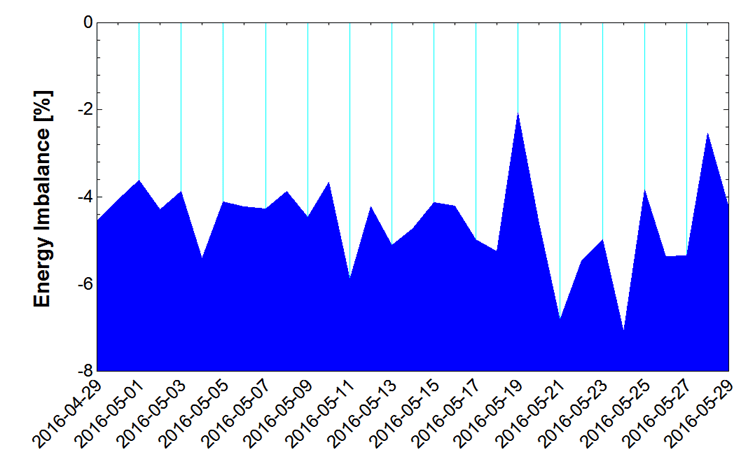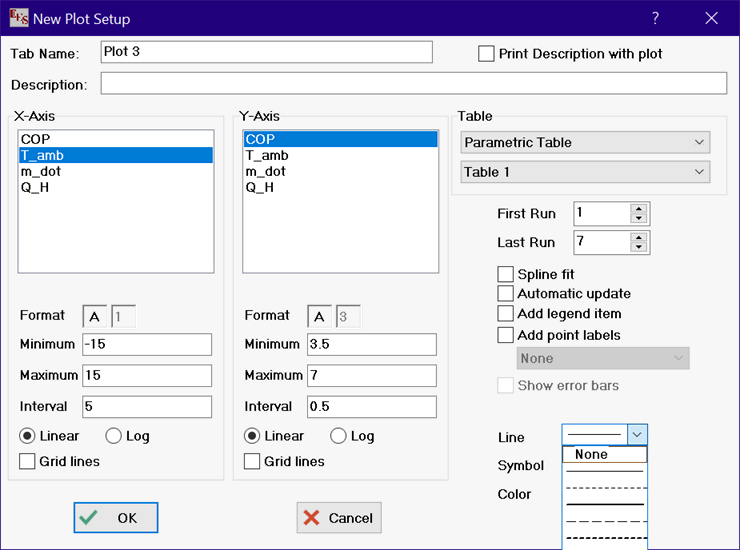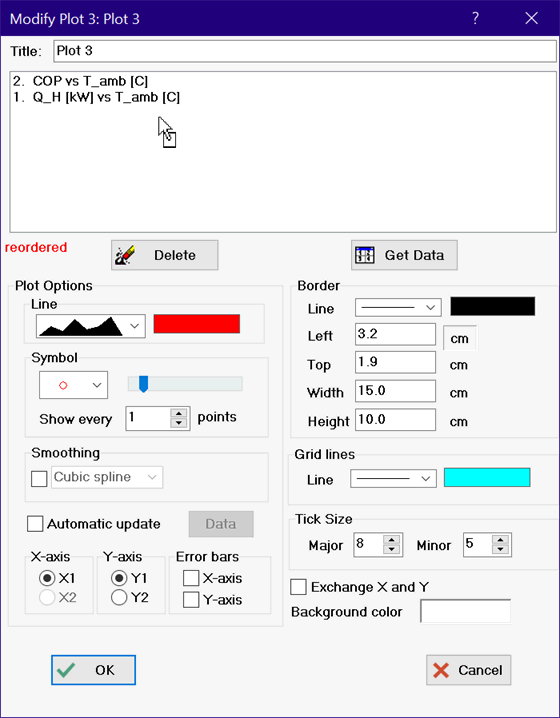Contents - Index

Area Plot

Area plots can be generated with the Professional license.  An area plot is similar to a line plot, but with the area below the plot line filled with a selected color as shown below.EES considers an area plot to be a type of X-Y (or line) plot.  For this reason, an area plot is constructed by selecting X-Y plot from the New Plot Window command in the Plots menu and then selecting the area plot option from the line drop down menu, as shown below.    Plot symbols normally are not needed with an area plot, so the plot symbol is set to None if an area plot is selected.An existing X-Y plot can be converted to an area plot using the Modify Plot command in the Plot menu.  Select the area plot from the line type drop in this dialog.

Note that an area plot can be only be successfully constructed if the values on the ordinate (X-axis) are continuously increasing or decreasing.  The Modify Plot dialog will disable the option to select an area plot if this is not the case.  Also, an area plot cannot use spline fits or smoothing.  If an area plot cannot be selected, you may be able to fill the desired area manually using the Polygon tool on the Plot. Tool bar.

Area plots are not transparent.  The most recently drawn plot will obscure part or all of the plots drawn previously if their areas overlap.  For this reason, it is often necessary to change the order in which plots are drawn.  This can be done in the Modify Plot dialog.  Plots are drawn in the order shown in the plot line list. The order can be changed by selecting a plot line that you want to move and dragging it to the desired location with the right mouse button depressed, as shown below. A 'drag' cursor will be displayed during this process. When the order is changed, reordered will be displayed below the left corner of the plot line list.  The reordering is not actually done until the Apply or OK buttons is clicked.